# G.3 Surface GREEN's Function

The main information needed to solve (G.14) are the surface GREEN's functions of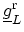and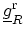. Using the recursive relation (H.10), equation (G.12) can be written as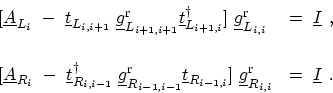(G.22)

If the potential does not vary in the left and right contacts and if the coupling between different layers are equal, then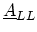and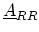become semi-infinite periodic matrices with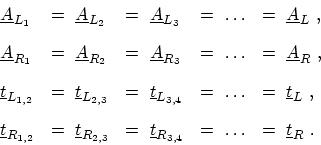(G.23)

Under this condition one obtains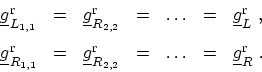(G.24)

Therefore, the surface GREEN's functions can be obtained by solving the quadratic matrix equations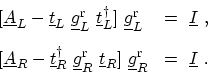(G.25)

These equations can be solved iteratively by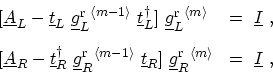(G.26)

whererepresents the iteration number. It should be noted that the solution to (G.25) is analytic if the dimension of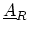is one. M. Pourfath: Numerical Study of Quantum Transport in Carbon Nanotube-Based Transistors